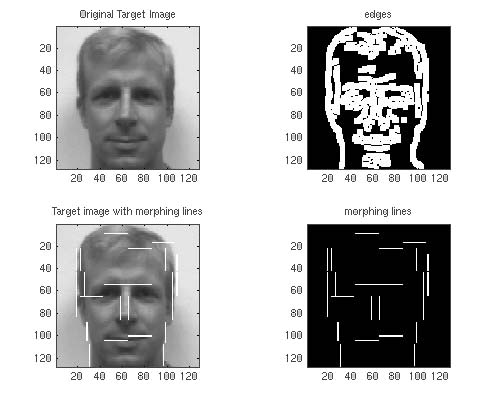## Edge Detection In Image Processing

Locating the boundaries of desired objects is another fundamental aspect of image processing. Helping to further segment images in order to locate and match objects, edge detection works by determining the gradient. This involves locating the discontinuities in intensity values throughout the image. There are many effective methods for doing this but just two of the most common methods, Sobel and Canny, will be discussed here.
Frequently used in many mathematical applications, the fundamental definition of the gradient is the derivative of one function in one direction to the function in multiple directions. In image analysis, the gradient is used to locate the change in brightness levels, indicating edges. To fully define the edge, the gradient is computed in both the horizontal and vertical directions. Edge detection algorithms, including the Sobel and Canny methods described below, incorporate finding the magnitude of this gradient. As mentioned, the Sobel method, named for Irwin Sobel, determines an approximate gradient of the image intensity function. It is based on convolving the image with a small and separable filter in both the horizontal and vertical directions. The
simplicity of this algorithm makes it attractive for being computationally inexpensive.

However, while it is proven to be effective, for some applications it can be too crude of an approximation. More effective than the Sobel operator, the Canny edge detection algorithm uses a multi-step algorithm to increase its robustness. The key steps within this method are first applying a Gaussian blur, next computing the intensity gradients, and finally tracing along the found edges and suppressing the non-maximum ones. The Gaussian blur is the convolving of an image using a Gaussian function which serves to reduce the image detail and noise. Next, the magnitude and directional derivative of the gradient is calculated to locate the edges. Once the edge has been located and the gradient directions known, the edge direction is related to a direction that can be traced in an image. Because pixels are arranged in a grid, when describing the directions of one pixel to surrounding pixels, there are only four directions that can be used. Zero degrees horizontally, ninety degrees vertically, forty-five degrees positively diagonal, and 135 degrees negatively diagonal. Therefore, the algorithm traces along the edge and rounds each edge direction to the closest applicable direction. To yield a more distinct edge, non-maximum suppression is applied. Again, the algorithm traces along the detected edge and it suppresses any point that it considers to be weak. To avoid image streaking, hysteresis is used. Hysteresis uses a high and a low threshold so any pixel that is higher than the high threshold is considered an edge pixel, and any pixel next to an already confirmed edge that has a value greater than the low threshold also remains as an edge.Edge Detection In Image Processing